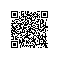# 通过SQL语句查看mysql数据库的大小

# use information_schema;

# select concat(round(sum(DATA_LENGTH/1024/1024),2),'MB') as data from TABLES;

# select concat(round(sum(DATA_LENGTH/1024/1024),2),'MB') as data from TABLES where table_schema='icinga';

# select concat(round(sum(DATA_LENGTH/1024/1024),2),'MB') as data from TABLES where table_schema='icinga' and table_name='aa';

yangzhimingg使用钉钉扫一扫加入圈子
+ 订阅